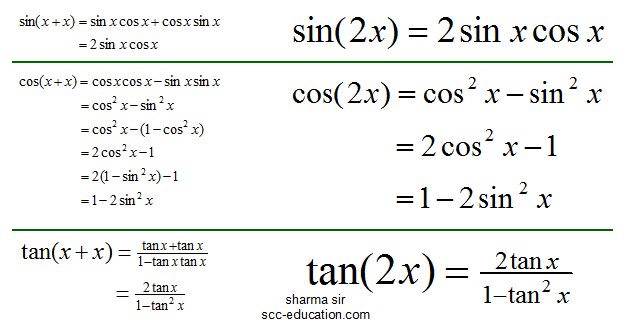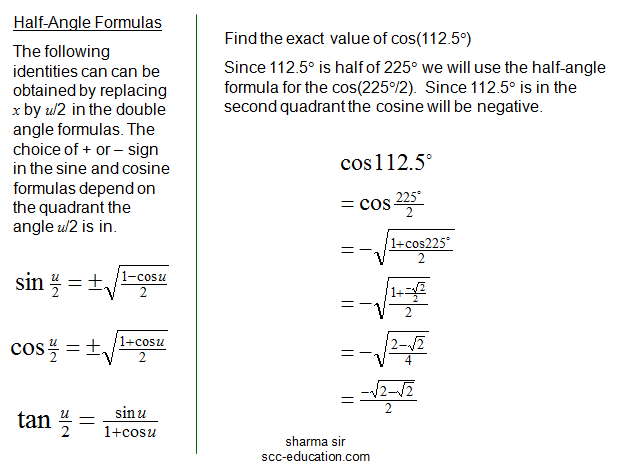SCC Education

Analytic Trigonometry Double-Angle, Half-Angle and Product-Sum Formulas

Analytic Trigonometry

Double-Angle, Half-Angle and Product-
Sum Formulas